# The sampSurf Package: Sampling Surface Simulation

## Overview of the sampSurf package

The sampSurf package can be used to construct sampling surface simulations for different areal sampling methods common to forestry and ecology. Areal sampling methods in general would include fixed-area plot methods, line intersect sampling, and various variable-radius plot sampling methods, the latter more commonly used in forestry. Most of these fall under the general probability proportional to size (pps) umbrella and have some form of inclusion zone associated with each individual in the population. The inclusion zone has well-defined area (sometimes called the inclusion area) and may be thought of simply as that zone within which a sample point could fall and select the associated individual. The sample point can be the center of a circular fixed-area plot, an actual point as in horizontal point sampling, or say, the midpoint of a line in line intersect sampling.

In general we are interested in determining the properties of various sampling methods mentioned above when applied to fixed objects such as standing trees or down logs. The sampSurf package will allow generation of log or tree populations within a fixed tract area. The surface generated from the intersection of inclusion zones applied to the individuals in the population for a given attribute (e.g., volume, number of individuals, etc.) are represented by the "sampSurf" class and can be displayed graphically. Estimator variance is directly associated with the sampling surface roughness, and so methods can also be compared visually.

There are several vignettes associated with the package that provide detailed explanation and examples of the design and use of the various components. A good place to start is with The sampSurf Package Overview vignette, which provides an overview of the class structure, etc. The vignettes are all available from the package index help page once the package has been installed and loaded using library(). Another overview of the package in the help system can be found by issuing package?sampSurf at the R command line. This help page will provide links to all of the built-in class structure, generics with associated methods, and helper functions. Running the examples (with R's example() command) will also provide some help in getting started.

sampSurf has classes and methods to support a number of different sampling protocols for both down logs and standing trees. The overall class structure is designed to support additions with relative ease, so more methods will appear in future releases (hopefully with some contributed by users). At the current release, there over 30 variants of sampling methods available in the package (e.g., each of the PDS methods below allows sampling with probability proportional to either volume, coverage or surface area)…

• #### Down Logs

1. Fixed-area plots: stand-up, sausage, and chainsaw protocols
2. Point-relascope sampling
3. Perpendicular distance sampling (PDS)
4. Omnibus PDS
5. Distance-limited PDS: canonical, hybrid and omnibus protocols
6. Distance-limited sampling: traditional and crude Monte Carlo protocols
• #### Standing Trees

1. Fixed-area plots
2. Horizontal point sampling
3. Horizontal point sampling with Monte Carlo subsampling (see below)
4. Horizontal line sampling
5. Critical height sampling
6. Importance critical height sampling
7. Antithetic importance critical height sampling
8. Paired antithetic importance critical height sampling

A recent addition to the package (in version 0.7-0) is the ability to sample down logs or standing trees for volume using Monte Carlo methods. These include crude Monte Carlo, importance sampling, control variate sampling, and antithetic versions of each. These are not areal methods, and apply directly to the stem objects themselves, so stem-based simulations can be conducted independently of an areal method. However, they can also be added to any areal method for a two-stage approach. For example, this has been incorporated in horizontal point sampling for standing trees.

Furthermore, as of version 0.7-2, the mirage method is available in addition to buffering for correction of boundary slopover. As in the buffer method, mirage correction is accomplished by running a simulation on a special mirage Tract subclass object. That is, for buffering, a bufferedTract is used, whereas for mirage, a mirageTract is used.

For more information on how each of these sampling and boundary correction methods is implemented within sampSurf please see package?sampSurf and the vignettes. The examples below also provide an illustration of some of these methods.

## sampSurf installation

sampSurf is now on CRAN. It is probably best to install it from there as the version here at R-Forge might not always be fully functional. However, if you do desire to use the version here, please read what follows as it may help.

On the project pages, you will note that you can install the package directly from R-Forge using…

install.packages("sampSurf", repos="http://R-Forge.R-project.org")

and can include the dep=TRUE argument if you want the packages that sampSurf is dependent upon to also be installed. Unfortunately, installing the dependencies in this way may be a little difficult. First, I believe that the dependencies are only searched for on R-Forge, and not CRAN (you could add more repos to the list to compensate). Since raster and rgl are both R-Forge projects, they will be found. But boot, for example, is evidently not on R-Forge and therefore R will complain that it can not find it. Second, the packages that are available on R-Forge could be in some intermediate stage of update, so it is better to install the required packages from CRAN, with what is known to be a stable release (including sampSurf). The dependencies are listed in the DESCRIPTION file for sampSurf, they are: sp, raster, rasterVis, and boot. In addition, both rgeos and rgl are suggested but not necessary unless you want to use the chainsaw method (or mirage boundary correction), or look at the sampling surfaces in 3D, respectively. Therefore, I would recommend that you use the normal install.packages() on all these packages so that you get the latest stable version from CRAN. Here is what I would try (in this order)…

install.packages("sp")
install.packages("raster")
install.packages("rasterVis")
install.packages("boot")
install.packages("sampSurf", repos="http://R-Forge.R-project.org")

Optional…
install.packages("rgeos")
install.packages("rgl")

The above should do it, while getting the correct stable releases from CRAN prior to installing sampSurf (again, it's probably best to install the CRAN version of sampSurf too). Please note that as of sampSurf version 0.6-6 (26-Nov-2012), rgeos has replaced gpclib due to the limited use license restriction on the latter (see this package on CRAN). Please see the instructions for installing rgeos if any problems arise.

### Enabling the R help system

One other thing that some R users may not be getting the full advantage of is the html help system. This really should be set up to work correctly in your `.Rprofile` file to get help via your web browser, using the local help file installation for each package (not just sampSurf). This will also enable you to see all of the vignette files for this package, which provide extensive documentation in PDF format. With the new dynamic help system that came in version 2.10.0, add these lines (with appropriate browser) to your `.Rprofile`

options(help_type = 'html')
options(browser='firefox')

That should do it on Linux, I have not tested this on other platforms, but it probably will work. Now when you do something like package?sampSurf at the R command line, you will get the response in your browser with hyper links to other useful pages.

### rgl Installation

On Linux, the rgl package requires system dependencies. The freeglut and mesa libraries must be installed. But in addition, the development versions of freeglut and libpng must also be install for rgl to run. On Fedora, these are `freeglut-devel` and `libpng-devel` which could be installed with `yum` prior to installing rgl within R. If you use windows or OS X, unfortunately I can not be of any help (but please let me know so I can post instructions here for others if there is anything extra one has to do to get rgl working on those systems).

### sampSurf package vignettes

As noted above, there are a number of package vignettes distributed with the package itself. These can also be downloaded here from the R-Forge versions if desired…

In addition, I have decided to remove some of the vignettes from the actual package structure itself because of the amount of space they consume, or the fact that they may be of limited interest to most users. Thus, the vignettes below are only available here at R-Forge…

Perhaps the most helpful introduction to sampSurf is found in the first vignette listed above. It provides more detail using extensive examples, on the organization, capabilites use of sampSurf.

## sampSurf examples

Here we present some very simple examples illustrating how to use a few of the main functions in the sampSurf package. The vignettes go into extensive detail concerning the use of the methods shown below. In addition, the online help system is quite thorough in documenting class structures, generic functions, and their methods. Therefore, we have provided just a very terse set of examples to show a little of the functionality of the package here.

In general, there are two main ways to create a sampling surface: (1) simple, usually "one-off" construction, or (2) progressive, by constructing intermediate elements that can be re-used for comparison of methods, etc. Both will be demonstrated below.

### Simple sampling surface construction

The first example shows how to create a sampling surface "on-the-fly," as it were, by hiding the intermediate steps used in building the surface. The only exception is that we always need some form of Tract object to be created ahead of time with the desired extents and resolution. This method is simple, but it does not easily allow for reuse of elements (e.g., the stem population, though it can be extracted if desired for reuse) in comparing other attribute surfaces, or different sampling methods.

The first component that is always required for a completed sampling surface is a Tract object with defined extents and resolution…

```R> require(sampSurf)
R> tract.m = Tract(c(x = 71, y = 71), cellSize = 0.5)     #meters
R> buffTract.m = bufferedTract(bufferWidth = 10, tract.m)
```

In the above example, we have first made a Tract object in metric (SI) units with no buffer that is one half hectare in area (71x71m), and has a grid resolution of 0.5m. The second step creates a buffered version with a 10m internal buffer. The two steps could, of course, be combined into one, but they are separated here for clarity. The internal buffer is generally set large enough that no inclusion zones will overlap the outer tract boundary; thus, eliminating concern for boundary slopover issues.

Next, simply run the sampSurf constructor to create an object of that class with the desired number of, in this case, downed logs (first argument). The sampling method to apply to the logs is passed in the iZone argument; e.g.,…

```R> sausageLen.ss = sampSurf(20, tract = buffTract.m, iZone = 'sausageIZ',
+                          plotRadius = 3, estimate = 'Length',
+                          buttDiams = c(20, 50), logLens = c(2, 8))
```
```Number of logs in collection = 20
Heaping log: 1,2,3,4,5,6,7,8,9,10,11,12,13,14,15,16,17,18,19,20,
```
```R> plot(sausageLen.ss, useImage = FALSE)
```Notice that you can specify the default log dimensions (e.g., buttDiams for the range in large-end log diameters) that will be used in creating the synthetic log population for this simulation. The plotRadius argument specifies the radius of the sausage-shaped inclusion zone to be associated with each log. The plot function shows a two-dimensional display of the sampling surface, with down logs plotted by default. The logs are created so that their midpoints (both longitudinal and radial) fall within the internal buffer of the bufferedTract object. Choosing a plot radius that keeps all of the inclusion zones within the tract for the longest possible log will ensure that all inclusion zones fit within the tract, eliminating boundary slopover issues. The constructor will warn you if any inclusion zones overlap the tract boundary.

A summary of the object is often useful…

```R> sausageLen.ss
```
```Object of class: sampSurf
------------------------------------------------------------
sampling surface object
------------------------------------------------------------

Inclusion zone objects: sausageIZ
Estimate: Length
Number of logs = 20

------------------------------------------------------------
object of class Tract
------------------------------------------------------------
Measurement units = metric
Area in square meters = 5041 (0.5041 hectares)

class       : bufferedTract
dimensions  : 142, 142, 20164  (nrow, ncol, ncell)
resolution  : 0.5, 0.5  (x, y)
extent      : 0, 71, 0, 71  (xmin, xmax, ymin, ymax)
coord. ref. : NA
data source : in memory
names       : surf
values      : 0, 1380.7419  (min, max)

Buffer width =  10
```
```R> summary(sausageLen.ss)
```
```Object of class: sampSurf
------------------------------------------------------------
sampling surface object
------------------------------------------------------------

Inclusion zone objects: sausageIZ
Measurement units = metric
Number of logs = 20
True log volume = 6.1546085 cubic meters
True log length = 86.33 meters
True log surface area = 77.289279 square meters
True log coverage area = 24.565998 square meters
True log biomass = NA
True log carbon = NA

Estimate attribute: Length
Surface statistics...
mean = 86.11307
bias = -0.21693013
bias percent = -0.25128012
sum = 1736383.9
var = 45264.698
st. dev. = 212.75502
cv % = 247.06472
surface max = 1380.7419
total # grid cells = 20164
grid cell resolution (x & y) = 0.5 meters
# of background cells (zero) = 16741
# of inclusion zone cells = 3423
```

Printing the object in the first line, displays the specification on the internal Tract object, which derives from RasterLayer in the raster package. Using summary on the object returns information about the sampling statistics for the object.

We can also display the surface nicely using the rgl package; take a minute to compare this to the two-dimensional display to see how the surface builds as inclusion zones overlap…

```R> require(rgl)
R> plot3D(sausageLen.ss)
R> par3d(zoom = 0.8)
```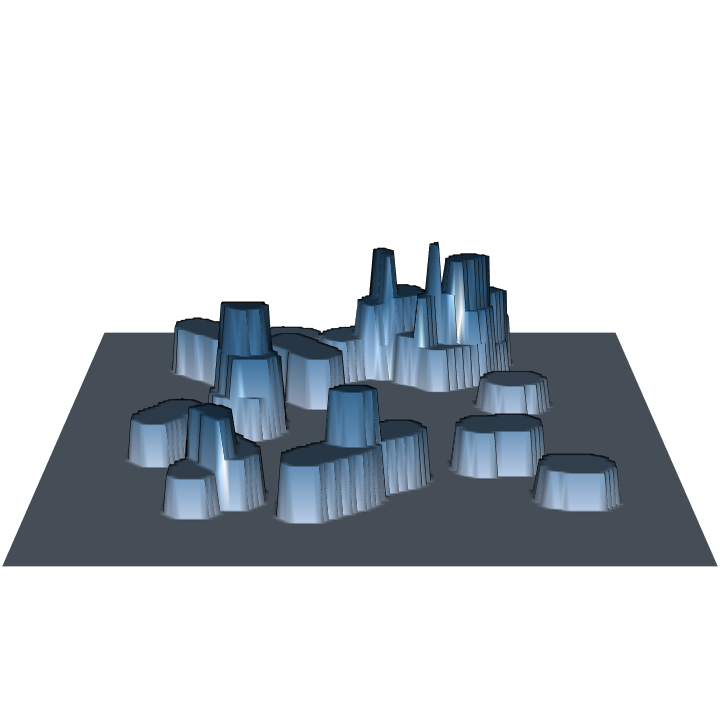Note that sampling for log length under the sausage sampling protocol of fixed-area plot sampling yields a constant height surface for each log, which varies between logs. This method is a probability proportional to length estimation method, and normally the heights would be constant for all logs under such a design. But sausage sampling is a little different in this respect because of the way the inclusion area is determined.

### Detailed sampling surface construction

In this section we will look at elaborating on what was done in the previous section by showing how a sampling surface can be built by creating most of the intermediate objects "by hand." (I say "most" here because InclusionZoneGrid objects are automatically created by the sampSurf constructor.) The basic steps are as follows…

1. Create a Tract object.
2. Create a population of synthetic stems in the form of logs or trees.
3. If need be, create an ArealSampling object with the specifications for the method.
4. Combine the stem population and the sampling method in the last two steps and form the population of InclusionZone objects.
5. Create the sampling surface from the collection in the previous step.
Some sampling methods or protocols do not require you to explicitly create an object that describes the sampling method in step 3; these include the fixed-area plot methods, where one simply specifies a plot radius. All other methods require information on design parameters as we will see below.

We will use the Tract object from the first example. In this example, we will look at another down coarse woody debris sampling method known as perpendicular distance sampling (pds). Start by creating a population of down logs…

```R> dLogs = downLogs(25, buffTract.m, buttDiams = c(25, 50), logLens = c(5, 10))
R> dLogs
```
```Object of class: downLogs
------------------------------------------------------------

------------------------------------------------------------
Container class object...
Units of measurement:  metric

Encapulating bounding box...
min       max
x 7.4339276 62.758264
y 8.7174424 62.270595

There are 25 logs in the population
Population log volume =  14.230931 cubic meters
Population log surface area =  174.73371 square meters
Population log coverage area =  55.585258 square meters
Average volume/log =  0.56923725 cubic meters
Average surface area/log =  6.9893486 square meters
Average coverage area/log =  2.2234103 square meters
Average length/log =  7.3228 meters
(**All statistics exclude NAs)
```
```R> plot(buffTract.m, gridColor = 'grey70')
```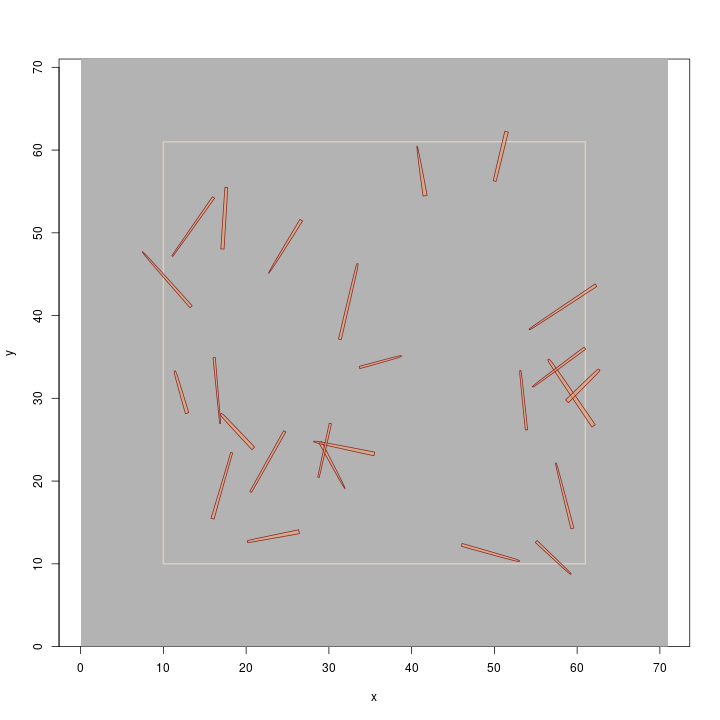We created a population of rather long, and large-diameter logs so they display nicely on the plot (remember, this is a metric example, and the buttDiams argument is in cm). The collection is created using the downLogs object constructor. The second line prints some summary information about the collection.

Next, since we know we want to use pds, we need to create an object that contains the information about that sampling method in the form of design parameters for the distance limit. This is most easily done in terms of a "Kpds factor" for the method…

```R> pds.m = perpendicularDistance(50, description = 'PDS parameters')
R> pds.m
```
```Object of class: perpendicularDistance
------------------------------------------------------------
PDS parameters
------------------------------------------------------------

ArealSampling...
units of measurement:  metric

perpendicularDistance...
kPDS factor = 50 per meter [or dimensionless] for volume [surface/coverage area]
volume [surface/coverage area] factor = 100 cubic meters [square meters] per hectare
```

The default is for metric units as we can see in the object summary. The associated volume, surface and coverage areas are determined for this particular Kpds factor and are also shown in the summary. I don't want to get into the structure of the package classes too much at this point, but the summary shows that the perpendicularDistance class is a subclass of ArealSampling, and the pertinent information for both classes is shown (i.e., the subclass inherits the units slot information from the superclass). With regard to the volume (surface or coverage area) factor, pds is a probability proportional to volume (surface or coverage area) sampling method, depending on which protocol is selected in the next step (where the inclusion zones are created). Therefore, each log counted on a sample point contributes, in this case, 100m3 (or 100m2) of volume (surface or coverage area) to the estimate.

The next step is to combine the collection of down logs and the appropriate sampling method parameters (the last two objects created above) and make the inclusion zones for each log. In the end, what we want is a collection of inclusion zones, one for each log, to match the dLogs object created above. The sampSurf package has "container classes" to help with this. For example, the dLogs object is a container of individual down log (class downLog) objects. Likewise, the following creates a container of all the individual inclusion zone objects. It does this by applying the perpendicularDistanceIZ constructor method to each log in the collection…

```R> dLogs.izs = downLogIZs(dLogs, iZone = 'perpendicularDistanceIZ', pds = pds.m,
+                        pdsType = 'volume',
+                        description = 'pds inclusion zones for dLogs')
R> dLogs.izs
```
```Container object of class: downLogIZs
------------------------------------------------------------
pds inclusion zones for dLogs
------------------------------------------------------------
There are 25 inclusion zones in the population
Inclusion zones are of class: perpendicularDistanceIZ
Units of measurement:  metric
Summary of inclusion zone areas in square meters...
Min.    1st Qu.     Median       Mean    3rd Qu.       Max.
24.594249  39.287022  52.811780  56.923725  73.104563 140.605338
var       SDev
607.335010  24.644168

Encapulating bounding box...
min       max
x 6.3594000 70.025991
y 5.0145724 63.960017
```
```R> plot(buffTract.m, gridColor = 'grey70')
```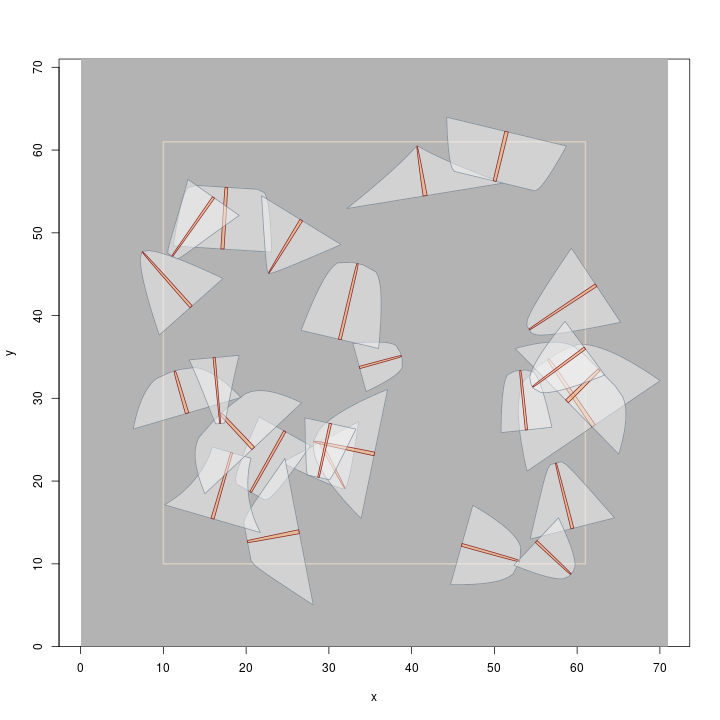Note that the downLogIZs constructor method creates the collection, and can work with any sampling method known to sampSurf that can be applied to sampling down logs (there's a companion method for standing trees as we will see in the next section). A summary of the collection is then printed showing some statistics on the inclusion zone areas (use hist on this object to display a histogram of the areas). Next, as a quick check to make sure all zones lie within the Tract object, the collection is plotted over the tract. Finally, we chose sampling with probability proportional to volume in this example (the pdsType argument). Therefore, the shape of the individual inclusion zones is an inflated version of each log's individual taper in terms of cross-sectional area along the stem (the integral of cross-sectional area is volume).

Of course now the last step is simply to create the sampling surface from the objects we have built in the previous steps. The sampling surface will be for volume in this case…
```R> dLogs.ss = sampSurf(dLogs.izs, buffTract.m)
```
```Number of logs in collection = 25
Heaping log: 1,2,3,4,5,6,7,8,9,10,11,12,13,14,15,16,17,18,19,20,21,22,23,24,25,
```
```R> summary(dLogs.ss)
```
```Object of class: sampSurf
------------------------------------------------------------
sampling surface object
------------------------------------------------------------

Inclusion zone objects: perpendicularDistanceIZ (with PP to: volume)
Measurement units = metric
Number of logs = 25
True log volume = 14.230931 cubic meters
True log length = 183.07 meters
True log surface area = 174.73371 square meters
True log coverage area = 55.585258 square meters
True log biomass = NA
True log carbon = NA

Estimate attribute: volume
Surface statistics...
mean = 14.21
bias = -0.020931233
bias percent = -0.14708266
sum = 286530.44
var = 825.22058
st. dev. = 28.726653
cv % = 202.15801
surface max = 151.23
total # grid cells = 20164
grid cell resolution (x & y) = 0.5 meters
# of background cells (zero) = 15534
# of inclusion zone cells = 4630
```
```R> plot(dLogs.ss, useImage = FALSE)
```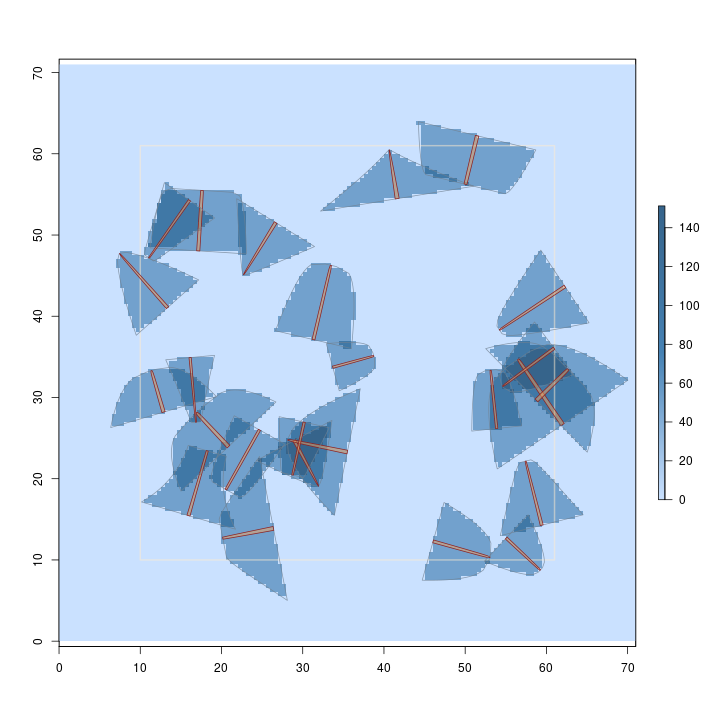Note in the summary that the method is unbiased. And, as in the first example, we can visualize the surface using rgl

```R> require(rgl)
R> plot3D(dLogs.ss)
R> par3d(zoom = 0.8)
```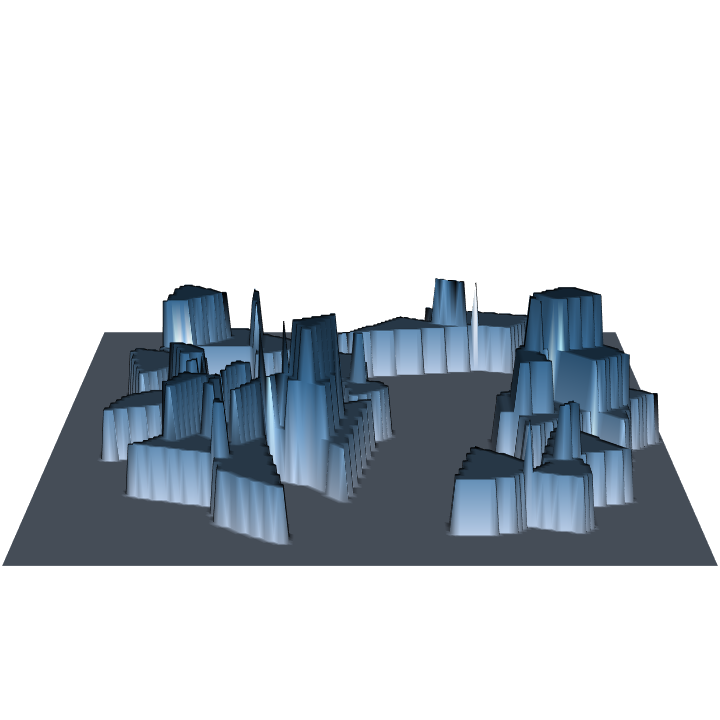Because we are sampling with probability proportional to volume, and estimating volume, the volume estimate for each log is the same and it gets spread evenly over its inclusion zone under pds. Also, since each log accounts for the same estimate (the volume factor), we get an even stair-step "heaping" of the surface where the inclusion zones intersect. This is the more normal case when you are sampling to estimate the "design attribute" that the method is optimized in terms of; the sausage protocol above is an exception to this rule.

### A final example

In the previous examples we have ignored standing trees and English units. Here we will rectify this. The steps presented below are the same as those outlined in the previous section, except they are applied to standing trees (and of course, the vignettes have much more detail on each step). You can look at (e.g., print, plot, hist, etc.) the objects created below to get more familiar with the package…

```R> tract.e = Tract(c(x = 208, y = 209), units = 'English', cellSize = 1) #feet here
R> buffTract.e = bufferedTract(bufferWidth = 30, tract.e)
R> sTrees = standingTrees(20, buffTract.e, dbhs = c(6, 15), heights = c(30, 50),
+                        units = 'English')
R> aGauge = angleGauge(baf = 20, units = 'English')
R> sTrees.izs = standingTreeIZs(sTrees, iZone = 'horizontalPointIZ',
+                              angleGauge = aGauge)
R> hps.ss = sampSurf(sTrees.izs, buffTract.e, estimate = 'Density')
```
```Number of trees in collection = 20
Heaping tree: 1,2,3,4,5,6,7,8,9,10,11,12,13,14,15,16,17,18,19,20,
```
```R> plot(hps.ss, useImage = FALSE)
```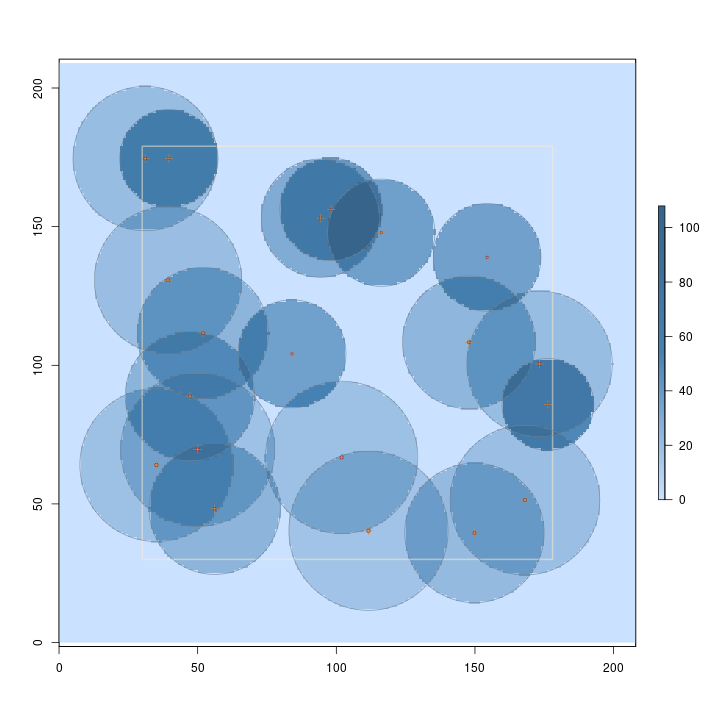```R> require(rgl)
R> plot3D(hps.ss)
R> par3d(zoom = 0.8)
```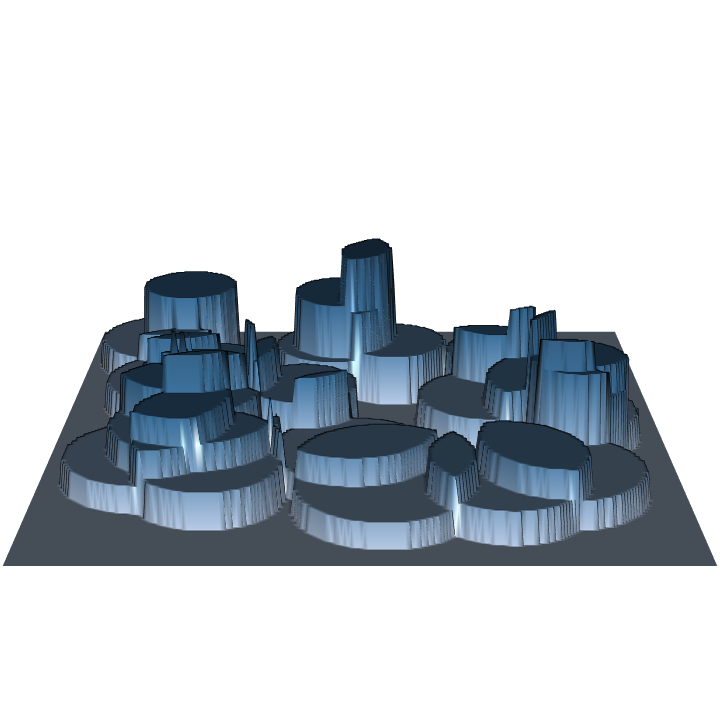Note in the figures that larger trees represent fewer trees per acre, and thus have lower surface height than smaller trees. Some sampling methods (or protocols for a given method) even have variable height surfaces within individual inclusion zones for each stem, perhaps you'll stumble across these in the use of this package.

### Summary

The examples presented assume some knowledge of the subject area with respect to areal sampling methods. Hopefully one can get an idea of what is involved in using the package and some uses it might be put to by comparing different (or new) methods on the sample stem populations. The vignettes provide much more detail and both they and the help pages have references to some of the more recent papers on both the sampling methods and the sampling surface concept. Please let me know if you've found sampSurf useful, or find any problems.